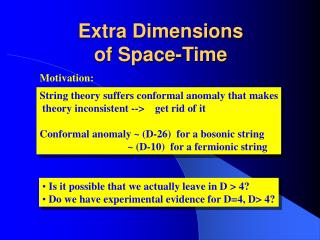DownloadDownload PresentationExtra Dimensions of Space-Time

# Extra Dimensions of Space-Time

Télécharger la présentation## Extra Dimensions of Space-Time

- - - - - - - - - - - - - - - - - - - - - - - - - - - E N D - - - - - - - - - - - - - - - - - - - - - - - - - - -
##### Presentation Transcript

1. Extra Dimensionsof Space-Time Motivation: String theory suffers conformal anomaly that makes theory inconsistent --> get rid of it Conformal anomaly ~ (D-26) for a bosonic string ~ (D-10) for a fermionic string • Is it possible that we actually leave in D > 4? • Do we have experimental evidence for D=4, D> 4?

2. Why don’t we see extra dimensions

3. Kaluza-Klein Approach compact space Pseudo-Euclidean space Minkowski space Metrics Fields Eigenfunctions of Laplace operator on internal space K d Masses K-K modes Radius of the compact space Couplings

4. Multidimensional Gravity Action K-K Expansion Newton constant Plank Mass Reduction formula

5. Low Scale Gravity 10 10 10 Modified Newton potential

6. Brane World Compact Dimensions Non-compact dimensions Kink soliton R Energy density brane Localization on the brane (Potential well) D4-brane New D4-brane SM Bulk Space-time of Type I superstring

7. graviton The ADD Model metric SM K-K gravitons Interactions with the fields on the brane The # of KK gravitons with masses Emission rate

8. Particle content of ADD model • (4+d)-dimensional picture: • (4+d)-dimensional massless graviton + matter • 4-dimensional picture • 1 massless graviton (spin 2) + matter • KK tower of massive gravitons (spin 2) • (d-1) KK spin 1 decoupling fields • KK tower of real scalar decoupling fields • KK tower of scalar fields (zero mode – radion) The SM fields are localized on the brane, while gravitons propagate in the bulk The “gravitational” coupling is

9. HEP Phenomenology New phenomena: graviton emission & virtual graviton exchange • KK states production bg LHC

10. HEP Phenomenology II • Virtual graviton exchange q - Spin=2 q SM Angular distribution

11. Randall-Sandrum Models D4-brane D4-brane Plank TeV Bulk Positive tension Negative tension Metric Matter warp factor graviton radion Perturbed Metric

12. Randall-Sandrum Model cont’d Brane 2 Brane 1 Wrap factor • Massless graviton • massive K-K gravitons • massless radion Hierarchy Problem ! • Massless graviton • massive K-K gravitons • massless radion

13. HEP Phenomenology The first KK graviton mode M ~ 1 TeV • Drell-Yan process • Excess in dijet process Exclusion plots for resonance production Excluded Excluded Run I Dj D-Y Run II D-Y Tevatron LHC

14. HEP Phenomenology II The x-section of D-Y production First KK mode First and subsequent KK modes Tevatron (M ~ 700 GeV) LHC (M ~ 1500 GeV)

15. HEP Phenomenology III Angular dependence LHC LHC

16. ED Conclusion • ADD Model • The MEW/MPL hierarchy is replaced by • For M small enough it can be checked at • modern and future colliders • For d=2 cosmological bounds on M are high (> 100 TeV), • but for d>2 are mild • Preictions of modification of the Newton’s law may be checked • RS Model • The MEW/MPL hierarchy is solved without new hierarchy • A large part of parameter space will be studied in future • collider experiments • With the mechanism of radion stabilization the model is viable • Cosmological scenarios are consistent (except the cosmological • constant problem)